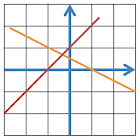Linear Equations

All Android applications categories

All Android games categories# Linear Equations

by: 33 8.6

8.6 Users
rating

## Screenshots

Description

This app is to calculate linear equations.

Features
• Solve 2 linear equations
• Solve 3 linear equations
• Load linear equation from CSV file
• Save linear equation to CSV file

Features in PRO version only:
1. Solve 3~9 linear equations
2. No Ads

Note :
1. For those who need support please email to me
Do NOT use either the feedback area to write questions, it is not appropriate and that is not guaranteed that can read them.

Tags : Linear equations, linear equation solver

from 33 reviews

"Great"

8.6